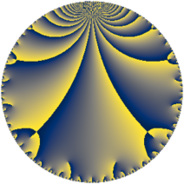# Properties

 Label 225.6.kLevel $225$ Weight $6$ Character orbit 225.k Rep. character $\chi_{225}(49,\cdot)$ Character field $\Q(\zeta_{6})$ Dimension $176$ Sturm bound $180$

# Learn more about

## Defining parameters

 Level: $$N$$ $$=$$ $$225 = 3^{2} \cdot 5^{2}$$ Weight: $$k$$ $$=$$ $$6$$ Character orbit: $$[\chi]$$ $$=$$ 225.k (of order $$6$$ and degree $$2$$) Character conductor: $$\operatorname{cond}(\chi)$$ $$=$$ $$45$$ Character field: $$\Q(\zeta_{6})$$ Sturm bound: $$180$$

## Dimensions

The following table gives the dimensions of various subspaces of $$M_{6}(225, [\chi])$$.

Total New Old
Modular forms 312 184 128
Cusp forms 288 176 112
Eisenstein series 24 8 16

## Trace form

 $$176q + 1378q^{4} - 100q^{6} - 878q^{9} + O(q^{10})$$ $$176q + 1378q^{4} - 100q^{6} - 878q^{9} + 586q^{11} - 1614q^{14} - 21058q^{16} + 8q^{19} + 3090q^{21} + 11490q^{24} - 17864q^{26} + 4690q^{29} + 4432q^{31} + 12726q^{34} - 46448q^{36} + 7670q^{39} + 50954q^{41} + 165064q^{44} - 91680q^{46} + 178818q^{49} - 75034q^{51} - 217802q^{54} + 89580q^{56} - 133270q^{59} - 46742q^{61} - 502544q^{64} - 90446q^{66} + 80214q^{69} + 31328q^{71} - 336740q^{74} + 48418q^{76} + 17450q^{79} + 252614q^{81} + 287550q^{84} + 158086q^{86} + 102876q^{89} + 131900q^{91} + 38250q^{94} + 352900q^{96} - 795910q^{99} + O(q^{100})$$

## Decomposition of $$S_{6}^{\mathrm{new}}(225, [\chi])$$ into newform subspaces

The newforms in this space have not yet been added to the LMFDB.

## Decomposition of $$S_{6}^{\mathrm{old}}(225, [\chi])$$ into lower level spaces

$$S_{6}^{\mathrm{old}}(225, [\chi]) \cong$$ $$S_{6}^{\mathrm{new}}(45, [\chi])$$$$^{\oplus 2}$$